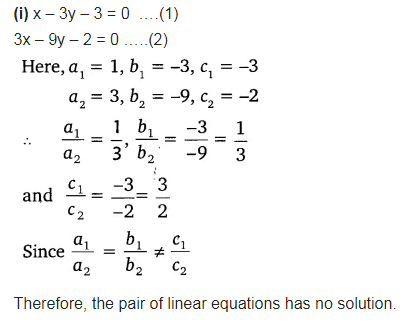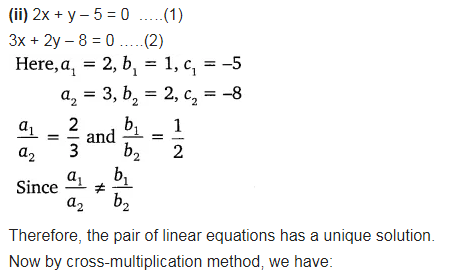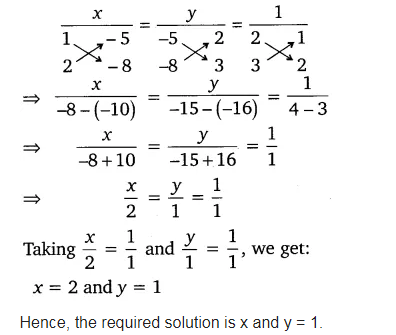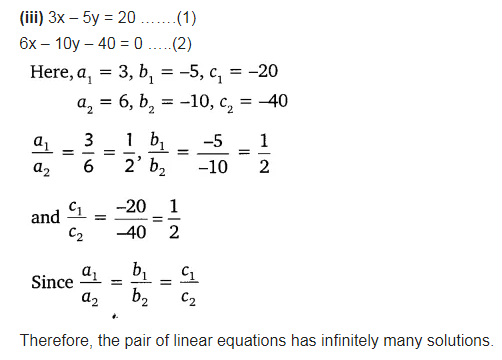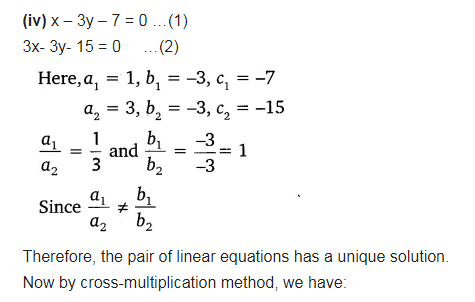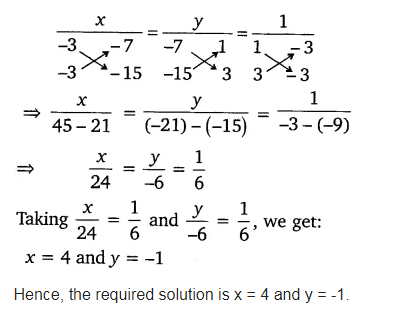# Which of the following pairs of linear equations has unique solution,

Which of the following pairs of linear equations has unique solution, no solution or infinitely many solutions. In case there is unique solution, find it by using cross-multiplication method.
(i) x – 3y – 3 = 0, 3x – 9y – 2 = 0
(ii) 2x + y = 5, 3x + 2y = 8
(iii) 3x – 5y = 20, 6x – 10y = 40
(iv) x – 3y – 7 = 0, 3x – 3y – 15 = 0